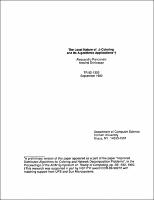## The Local Nature of $\Delta$-Coloring and Its Algorithmic Applications##### Abstract
Given a connected graph $G$ = ($V,E$) with $\mid$V$\mid$ = $n$ and maximum degree $\Delta$ such that $G$ is neither a complete graph nor an odd cycle, Brooks' theorem states that $G$ can be colored with $\Delta$ colors. We generalize this as follows: let $G$ - $v$ be $\Delta$-colored; then, $v$ can be colored by considering the vertices in an $O$(log$_{\Delta} n$) radius around $v$ and by recoloring an $O$(log$_{\Delta} n$) length "augmenting path" inside it. Using this, we show that $\Delta$-coloring $G$ is reducible in $O$(log$^{3}$ $n$/log $\Delta$) time to ($\Delta$ + 1)-vertex coloring $G$ in a distributed model of computation. This leads to fast distributed algorithms and a linear-processor $NC$ algorithm for $\Delta$-coloring.
1992-09
##### Publisher
Cornell University
##### Keywords
computer science; technical report
##### Previously Published As
http://techreports.library.cornell.edu:8081/Dienst/UI/1.0/Display/cul.cs/TR92-1303
technical report